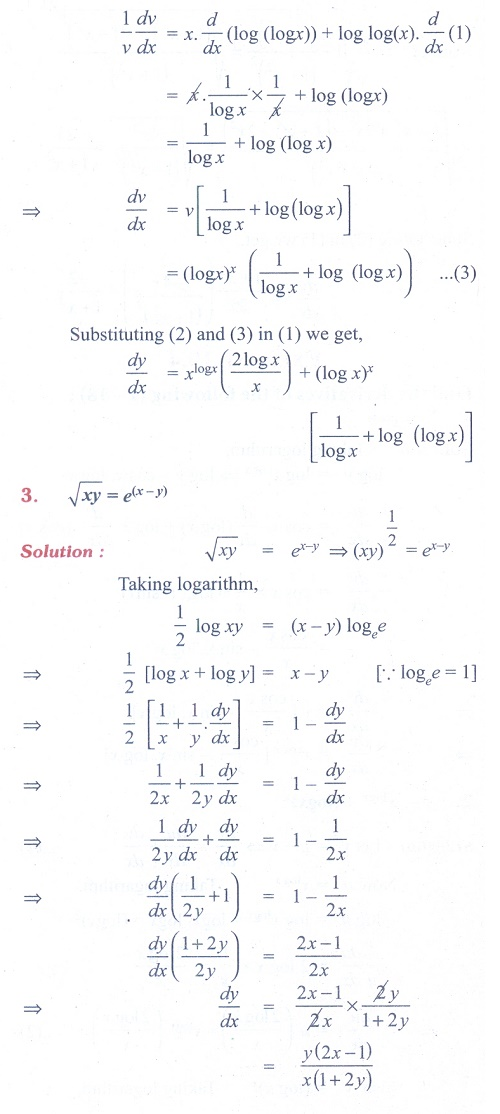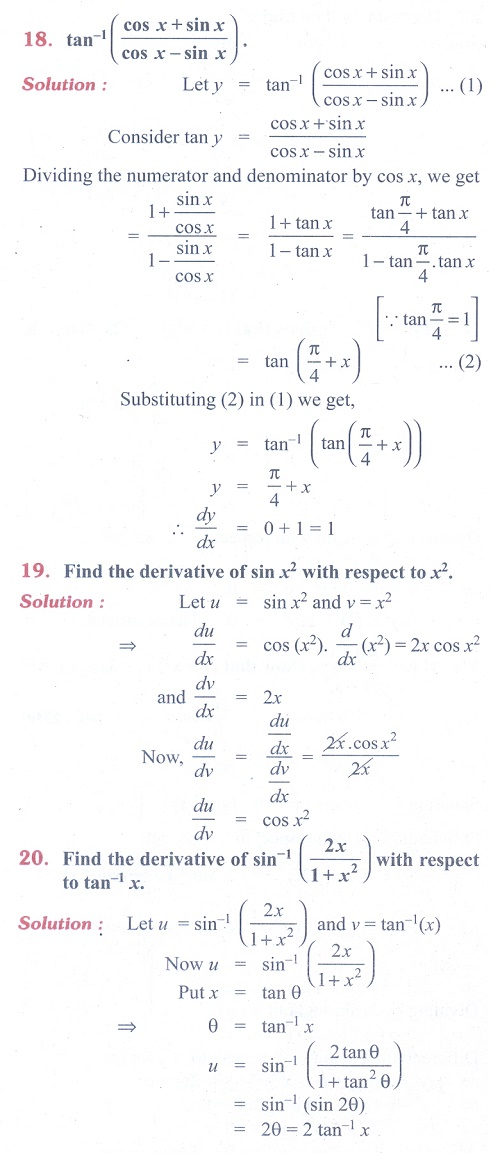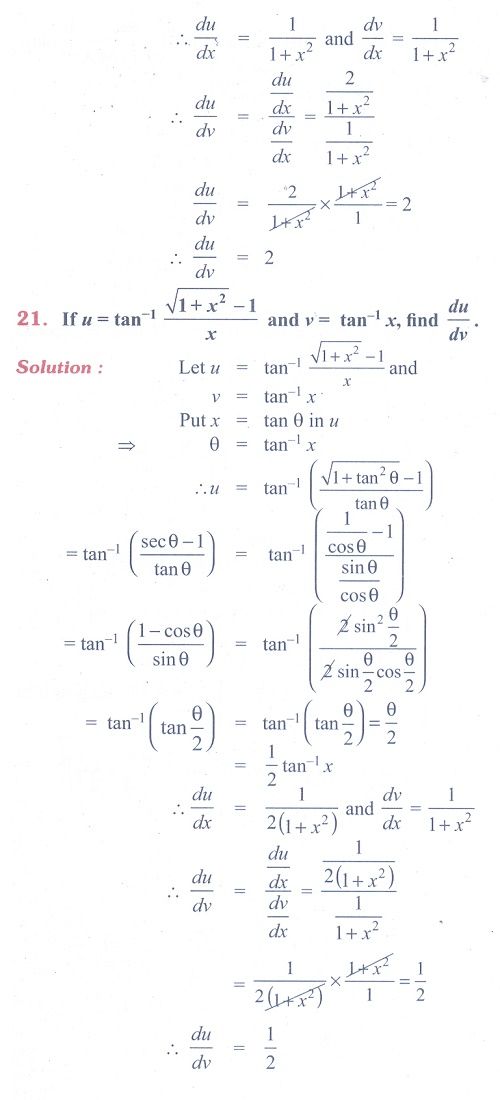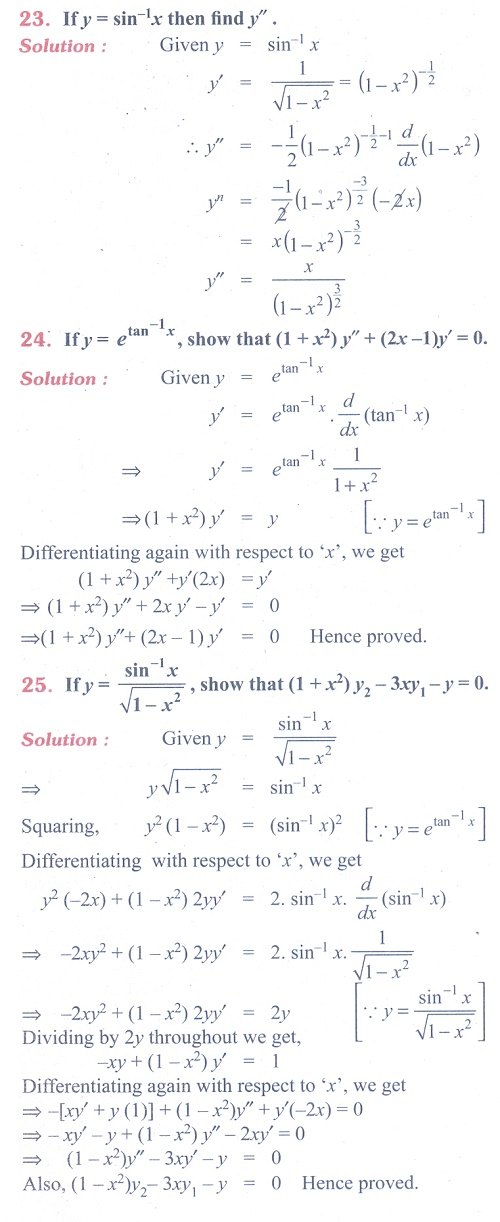Home | | Maths 11th std | Exercise 10.4: Higher order Derivatives

# Exercise 10.4: Higher order Derivatives

Maths Book back answers and solution for Exercise questions - Mathematics : Differential Calculus- Differentiability and Methods of Differentiation: Higher order DerivativesTags : Problem Questions with Answer, Solution | Differential Calculus | Mathematics , 11th Mathematics : UNIT 10 : Differential Calculus: Differentiability and Methods of Differentiation
Study Material, Lecturing Notes, Assignment, Reference, Wiki description explanation, brief detail
11th Mathematics : UNIT 10 : Differential Calculus: Differentiability and Methods of Differentiation : Exercise 10.4: Higher order Derivatives | Problem Questions with Answer, Solution | Differential Calculus | Mathematics